# Difference Between Hydrostatic and Osmotic Pressure

## Main Difference – Hydrostatic vs Osmotic Pressure

Pressure is defined as the force applied on a unit area perpendicularly. Hydrostatic pressure and osmotic pressure are two classifications of pressure that are in relation with fluids. The main difference between hydrostatic pressure and osmotic pressure is that hydrostatic pressure is found in any type of homogenous fluid whereas osmotic pressure is not found in pure solutions.

### Key Areas Covered

1. What is Hydrostatic Pressure
– Definition, Characteristics, Equation and Calculation
2. What is Osmotic Pressure
– Definition, Characteristics, Equation and Calculation
3. What is the Difference Between Hydrostatic and Osmotic Pressure
– Comparison of Key Differences

Key Terms: Homogenous, Hydrostatic Pressure, Osmotic Pressure, Pressure, Van’t Hoff Equation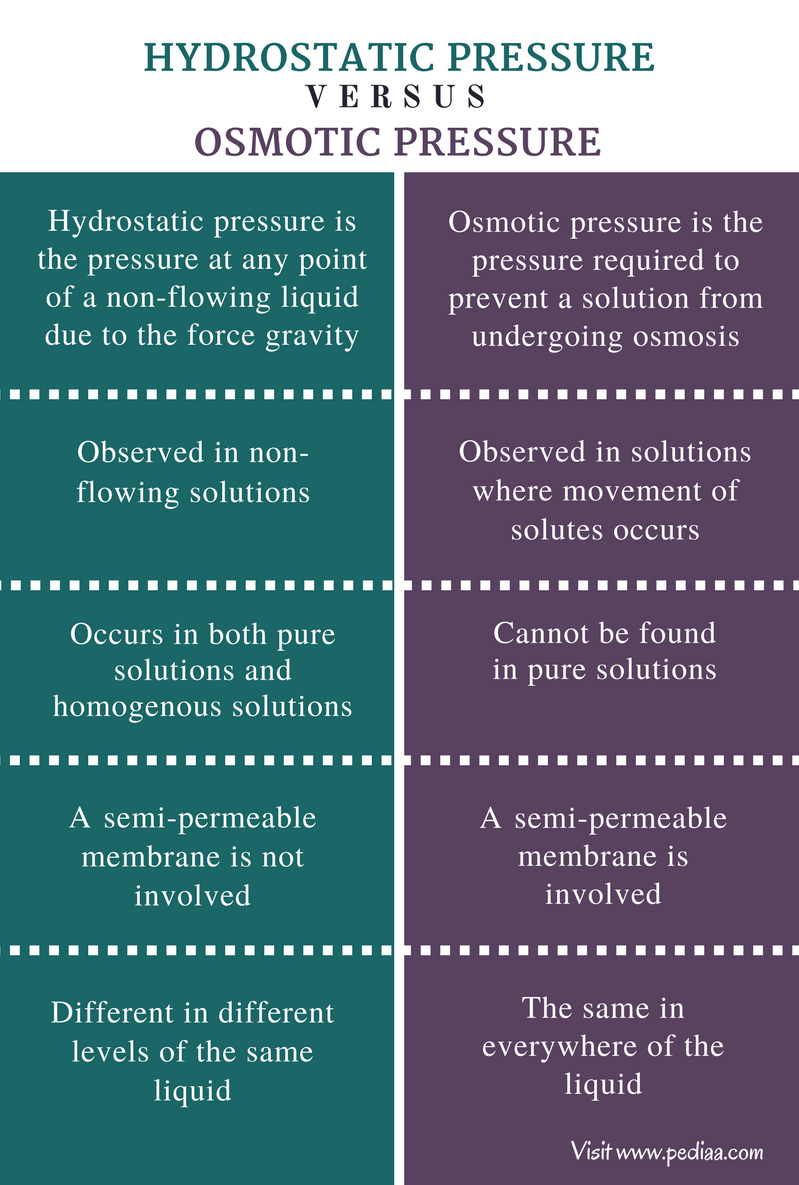## What is Hydrostatic Pressure

Hydrostatic pressure is the pressure at any point of a non-flowing liquid due to the force of gravity. Consider a jar of water. The pressure at the surface of the water is atmospheric pressure. That is the pressure applied on the water by the atmosphere. But if we consider a point at the middle of the water in that jar, the pressure in that point is different from that of the surface. That is because the water above that point also applies a pressure on that point due to gravity.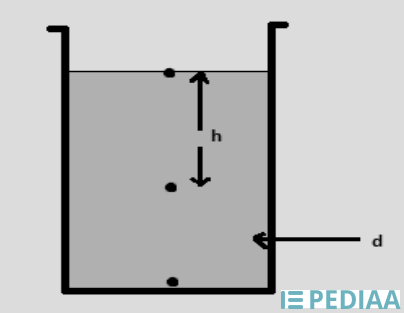Figure 1: A jar or container showing the density (d) of the water and the depth to the middle point of the jar

The above image shows a jar of water. There are three points marked on it. The pressure at the point on the surface of the water is atmospheric pressure. This atmospheric pressure can be given as π. The point in the middle is at a depth of h from the surface. The pressure applied by a liquid is given as

P = hdg

Where,

P is the pressure applied

h is the depth or the height of the liquid body

d is the density of the liquid

g is the gravity

Therefore, the pressure on the middle point in above image can be given as,

Ptotal  =  π + hdg

The pressure on the bottom of the jar is,

Ptotal  =  π + 2 hdg

Therefore, the hydrostatic pressure at different points of the same liquid is different. But the hydrostatic pressure at points in the same level of the same liquid is the same.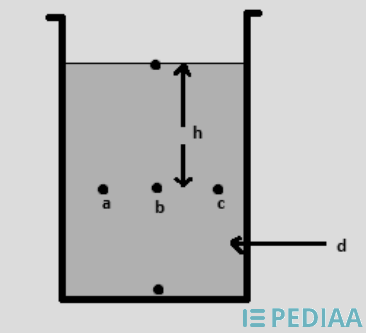Figure 2: A jar of water showing three points located at the same level.

In the above image, “a”, “b”, and “c” are located on the same level. Therefore, the pressure at each point would be same. This hydrostatic pressure causes different velocities in water flow at different points of the same liquid. This phenomenon is shown in the below diagram.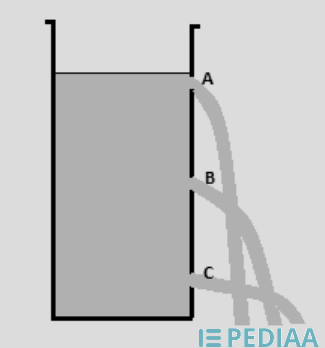Figure 3: Different velocities of water at different levels.

In the above image, A, B and C are holes located at different levels in the same jar of water. The highest velocity is observed at point C. This is because a higher pressure is applied to the point C. The lowest velocity is observed at A since only the atmospheric pressure is applied to that point.

## What is Osmotic Pressure

The osmotic pressure is the pressure required to prevent a solution from undergoing osmosis. The basic requirement for this osmotic pressure to occur is the presence of at least two different solutions that are separated from each other by a semi-permeable membrane.

A semi-permeable membrane is a very thin membrane that only allows solute molecules to pass through. Therefore, the solute molecules pass through it until the concentration of the solute becomes the same in both solutions. The solvent is always moved from high concentration to low concentration.  Osmotic pressure is the force needed to stop this movement.

Osmotic pressure also can be abbreviated mathematically. The equation that gives the osmotic pressure is called Van’t Hoff equation.

Π    =     nRT / V

Where

Π is the osmotic pressure

n is the number of moles of solute

R is the universal gas constant

T is the absolute temperature

V is the volume of the solution

However, n/V is equal to the molarity of the solution. For example, a solution containing KCl (1.00M) at 298K temperature may have an osmotic pressure of,

Π       =       nRT / V

=       MRT

=       1.00 mol/L  x   0.0821 L atm /(mol. K)  x   298 K

=       24.466 atm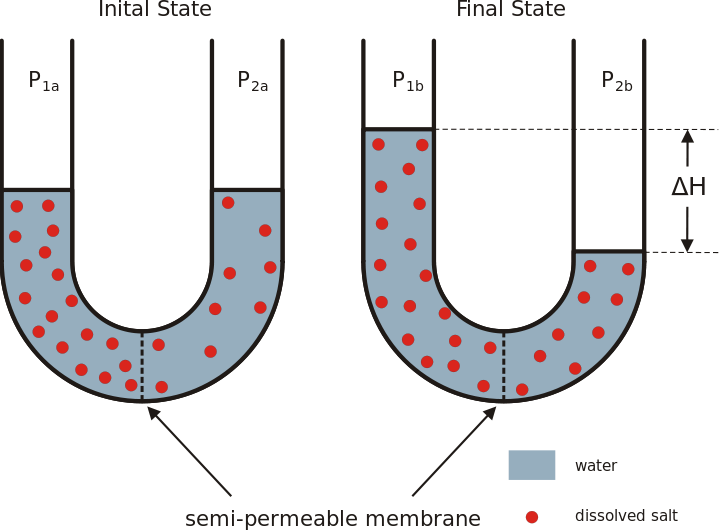Figure 4: Osmotic pressure causes the final concentrations of the two solutions to become equal.

## Difference Between Hydrostatic and Osmotic Pressure

### Definition

Hydrostatic Pressure: Hydrostatic pressure is the pressure at any point of a non-flowing liquid due to the force gravity.

Osmotic Pressure: Osmotic pressure is the pressure required to prevent a solution from undergoing osmosis.

### Movement of the Solute

Hydrostatic Pressure: Hydrostatic pressure is observed in non-flowing solutions.

Osmotic Pressure: Osmotic Pressure is observed in solutions where movement of solutes occurs.

### Purity of the Solution

Hydrostatic Pressure: Hydrostatic pressure occurs in both pure solutions and homogenous solutions.

Osmotic Pressure: Osmotic pressure cannot be found in pure solutions.

### Semi-permeable Membrane

Hydrostatic Pressure: In hydrostatic pressure, a semi-permeable membrane is not involved.

Osmotic Pressure: In osmotic pressure, a semi-permeable membrane is involved.

### Pressure at Different Levels

Hydrostatic Pressure:  Hydrostatic pressure is different in different levels of the same liquid.

Osmotic Pressure: Osmotic pressure is the same in everywhere of the liquid; thus, it is calculated considering the whole system.

### Conclusion

Hydrostatic pressure and osmotic pressure are two types of pressure that refer to pressure in liquids. The main difference between Hydrostatic Pressure and Osmotic Pressure is that Hydrostatic Pressure is found in any type of homogenous fluid whereas Osmotic Pressure is not found in pure solutions.

##### References:

1.”Osmotic pressure: Definition and formula.” Study.com. Study.com, n.d. Web. Available here. 04 July 2017.
2.”Colligative Properties: Osmotic Pressure.” Chemistry LibreTexts. Libretexts, 16 Feb. 2017. Web. Available here. 04 July 2017.

##### Image Courtesy:

1. “Osmose en” By © Hans Hillewaert (CC BY-SA 3.0) via Commons Wikimedia